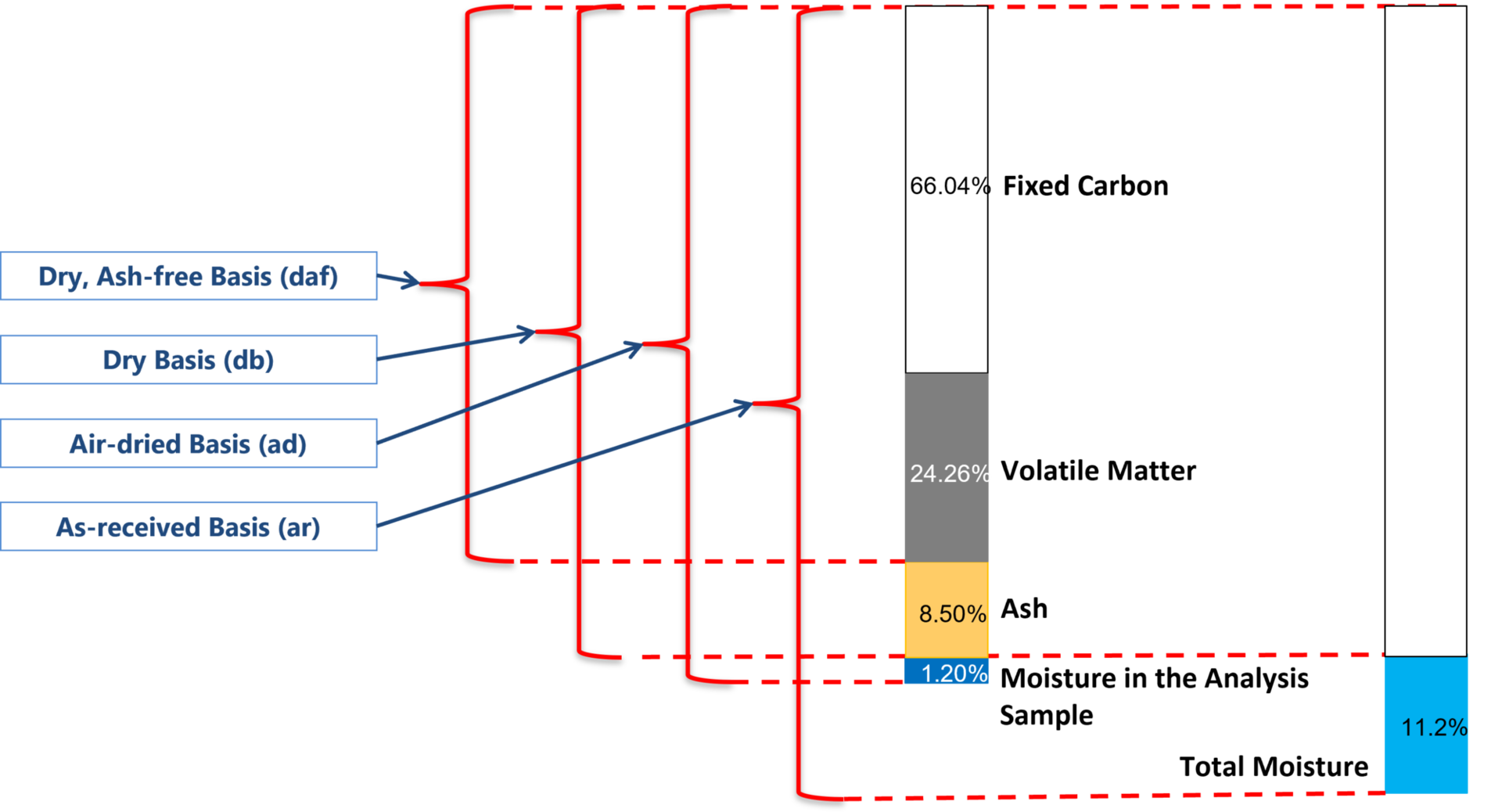Take the next step.

Request a quote for our quality-assured analysis and sampling services, and we will get back to you in the next couple of business days.

Get in touch with us.

Have questions or comments about our water quality services? Please send us a message and we will get in touch with you soon.

# Coal basis conversion

### Coal basis introduction

Coal analysis can be reported to a range of different "basis" depending on the purpose of the analysis. For example, "as received" or "as sampled" basis is used to understand the concentration of analytes in a coal including all the surface moisture. In contrast, reporting results on a "dry, ash-free" basis can be useful to understanding the rank of the coal, particularly when reviewing Volatile Matter, Hydrogen or Carbon concentrations. There may also be commercial reasons why a particular moisture basis is required, notably when a coal contract is written to a specified moisture basis, for example "2.5% moisture" basis

When the basis changes the associated analytical results will also change. Consequently, when comparing results it is important to compare them on the same basis.### Different coal bases

There are a range of commonly used coal bases, each of which has a prescribed abbreviation. Bases include:

• As Received (or as sampled) Basis (ar) – the analysis are reported based on the total moisture of the sample. That is in the as-shipped state.
• Air Dry Basis (ad) – the condition in which the moisture content of the analytical sample has come into equilibrium with the humidity of the laboratory atmosphere.
• Dry Basis (d) – the condition in which the sample is assumed to be completely free of moisture.
• Dry, Ash-Free Basis (daf) – a hypothetical condition for which the sample is assumed to be completely free of moisture and ash. This allows a judgement based on only the organic component of the coal. (Commonly used for assessing calorific value or coal rank).
• Dry, Mineral Matter Free Basis (dmmf) – the ash concentration is converted mathematically to a theoretical mineral concentration.

##### Converting from one coal basis to another coal basis

The relationship between the various bases is provided in the figure below.The general equation for conversion from one moisture basis to another moisture basis is as follows:

General Equation:

100 – moisture basis required . analyte result as analyzed = analyte at required moisture basis
100 – moisture basis as analyzed

Example 1: You have a set of air-dried results where the as-analyzed Moisture is 2.0% (ad) and Ash is 12.0% (ad). For a contract you are required to report the Ash to a Moisture basis of 3.0% basis.

100 – Moisture (required basis) . Ash result (ad) = Ash result (at required basis)

(100 – 3.0) . 12.0 = 11.9% (ad)
(100 – 2.0)

Example 2: You are required to report an Ash value to Total Moisture (ar) basis where the air dried Moisture is 2.0% (ad), the air dried Ash is 12.0% (ad) and the Total Moisture is 10% (ar).

100 – Moisture (ar) . Ash result (ad) = Ash (ar)

(100 – 10.0) . 12.0 = 11.0% (ar)
(100 – 2.0)

Example 3: You analyzed a sample which has the following characteristics: Moisture 2.0% (ad), Ash 12.0% (ad) and Volatile Matter 29.5% (ad). You are required to calculate the Volatile Matter to a dry, ash free (daf) basis.

100 – Moisture (daf) + Ash (daf) . Volatile Matter (ad) = Volatile Matter (daf)

(100 – (0 + 0)) . 29.5 = 34.3 (daf)
(100 – (2.0 + 12.0))

The numerators and denominators used to convert a result from one basis to another basis are provided in the table below. These are multiplied by the existing analyte result to produce the analyte result at the required basis.

Existing basis Desired basis
As received Air Dried Dry Dry Ash Free
100 – M(ar)
100
100 – M(ar)
100
100–(M(ar)+A(ar))
Air Dried 100 – M(ar)
100
100
Dry 100 –M(ar)
100
100
100
100–A(d)
Dry Ash Free 100-(M(ar)+A(ar))
100
100
100 – A(d)
100

#### Related services#### Moisture in Coal

For information on how to determine in-situ moisture, refer to Moisture in Coal.